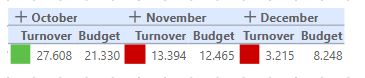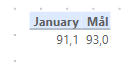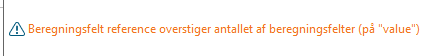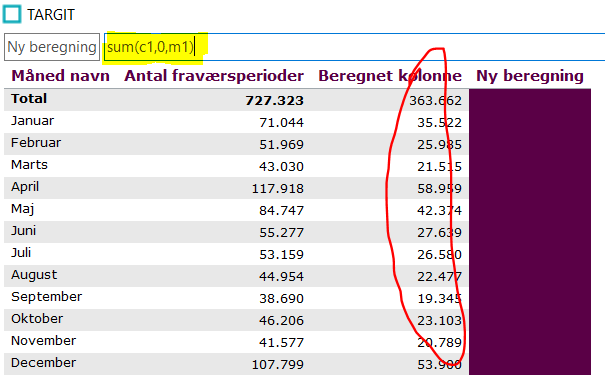# Color agent based on measure values

Hello,

Would it be possible to base color agents based on measure values? For example, I have a table with Column A(measure 1) and column B(measure 2). I want to add a color agent to show an arrow whenever the value of measure 1 is above measure 2. I know I can add a custom calculation to calculate the difference, and base the color agent on that. But I want to add the color agent in column A.

Would this be possible?

• Yes,

You create a color agent on measure1 with code

`Value > sum(d1,0,m2)`

Alternatively I like to specify what is happening in the color agent by naming the references like this:

If for instance m1 was YTDSales and m2 is YTDLastYearSales

`YTdSales:(sum(d1,0,m1)) > YTDLastYearSales:(sum(d1,0,m2))`
• This works for me but not if I have more than one month like in this example. I would like November to be green...I probably have to modify the code, but how?

`Value > sum(d1,0,m2)`
• Hi Michael,

Try with

`value > sum(0, 0, m2)`

BR / Ole

• Great Ole, thank you. That worked wonders for me...

But now another problem has occured. Apparently these codes aren't my best asset ;-)

I have an almost identical task here so I thought I was able to use the same code.

I want a color agent showing whether the January result is better or worse than the target of 93,0The difference to the last task is that "Mål" is a calculation and not a measure. Maybe that makes a difference to how the code should appear?

I get this error when trying to apply the code:• Hi Michael

If it is a calculated column you need to refer to it as c1, c2, c3 etc. instead of m1, m2, m3.

So the syntax might be: value > sum(c1,0,m1)

If you click at "Advanced calculations":And try to make a new calculation, you can click at the column/measure you want to refer to. Then you get the correct syntax. And then you can use this syntax in your colouragent. (and just delete the new calculation again)